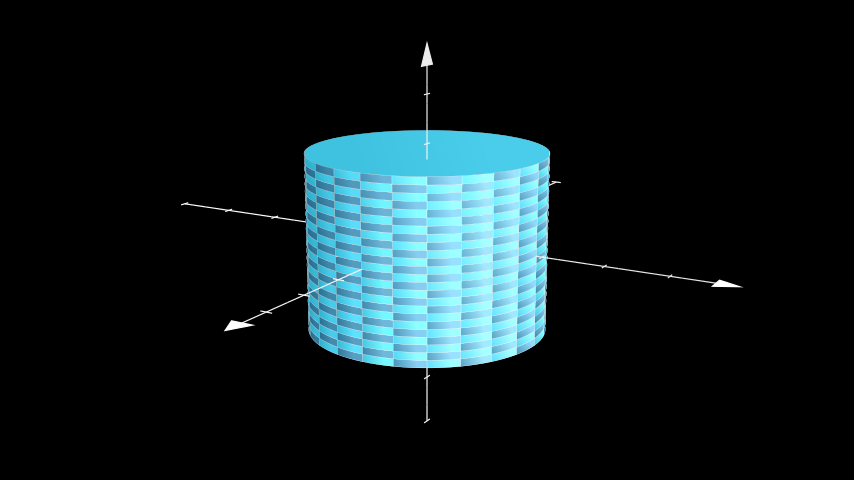# Cylinder¶

Qualified name: `manim.mobject.three\_dimensions.Cylinder`

class Cylinder(radius=1, height=2, direction=array([0., 0., 1.]), v_range=[0, 6.283185307179586], show_ends=True, resolution=(24, 24), **kwargs)[source]

A cylinder, defined by its height, radius and direction,

Examples

Example: ExampleCylinder```from manim import *

class ExampleCylinder(ThreeDScene):
def construct(self):
axes = ThreeDAxes()
self.set_camera_orientation(phi=75 * DEGREES, theta=30 * DEGREES)
```
Parameters
• radius (`float`) – The radius of the cylinder.

• height (`float`) – The height of the cylinder.

• direction (`numpy.array`) – The direction of the central axis of the cylinder.

• v_range (`Sequence[float]`) – The height along the height axis (given by direction) to start and end on.

• show_ends (`bool`) – Whether to show the end caps or not.

Methods

 `add_bases` Adds the end caps of the cylinder. `func` Converts from cylindrical coordinates to cartesian. `get_direction` Uses `shoelace_direction()` to calculate the direction. `set_direction`

Attributes

 `animate` Used to animate the application of a method. `animation_overrides` `depth` The depth of the mobject. `height` The height of the mobject. `width` The width of the mobject.

Adds the end caps of the cylinder.

func(u, v)[source]

Converts from cylindrical coordinates to cartesian. :param u: The height. :type u: `float` :param v: The azimuthal angle. :type v: `float`

get_direction()[source]

Uses `shoelace_direction()` to calculate the direction. The direction of points determines in which direction the object is drawn, clockwise or counterclockwise.

Examples

The default direction of a `Circle` is counterclockwise:

```>>> from manim import Circle
>>> Circle().get_direction()
'CCW'
```
Returns

Either `"CW"` or `"CCW"`.

Return type

`str`